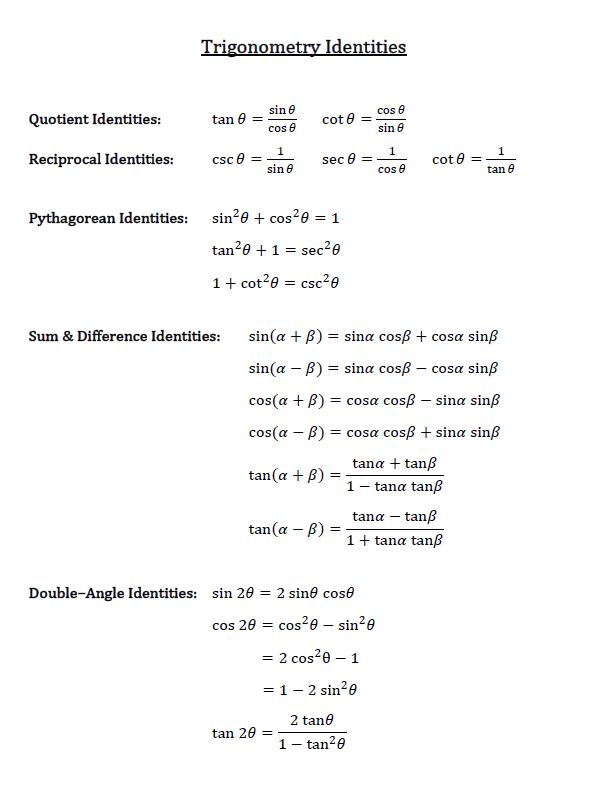# Double-angle identities

0/0
0/10
##### Examples
###### Lessons
1. Express each of the following in terms of a single trigonometric function:
1. $14 \sin 6x \cos 6x$
2. $\cos {9 \over 2} x \sin {9 \over 2 } x$
3. $\cos^25A - \sin^25A$
4. $3 - 6 \cos^2 8x$
5. $\frac{6 \tan 5x}{\tan^25x - 1}$
2. Prove identities
1. $\frac{\sin 6x}{ 1 + \cos 6x} =\tan 3x$
2. $\cot x - \tan x= \frac{4 \cos^2x - 2}{\sin 2x}$
3. $\frac{\sin 4x - \sin 2x}{\cos 4x + \cos 2x} = \tan x$
4. $\frac{\cot x - \cos x}{ 1 - \sin x} = \frac{\sin2x}{1 - \cos2x}$
3. Given $\tan A = -2,$ where ${3 \pi \over 2 } \leq A \leq 2\pi,$
find the exact value of:
i)
$\sin 2A$
ii)
$\sec 2A$
iii) $\tan 2A$
###### Free to Join!
StudyPug is a learning help platform covering math and science from grade 4 all the way to second year university. Our video tutorials, unlimited practice problems, and step-by-step explanations provide you or your child with all the help you need to master concepts. On top of that, it's fun - with achievements, customizable avatars, and awards to keep you motivated.
• #### Easily See Your ProgressWe track the progress you've made on a topic so you know what you've done. From the course view you can easily see what topics have what and the progress you've made on them. Fill the rings to completely master that section or mouse over the icon to see more details.
• #### Make Use of Our Learning Aids###### Practice Accuracy

See how well your practice sessions are going over time.

Stay on track with our daily recommendations.

• #### Earn Achievements as You LearnMake the most of your time as you use StudyPug to help you achieve your goals. Earn fun little badges the more you watch, practice, and use our service.
• #### Create and Customize Your AvatarPlay with our fun little avatar builder to create and customize your own avatar on StudyPug. Choose your face, eye colour, hair colour and style, and background. Unlock more options the more you use StudyPug.
###### Topic Notes
In this lesson, we will learn how to make use of the double-angle identities, a.k.a. double-angle formulas to find the sine and cosine of a double angle. It's hard to simplify complex trigonometric functions without these formulas.

## Using double angle identities in trigonometry

Identities in math shows us equations that are always true. There are many trigonometric identities (Download the Trigonometry identities chart here ), but today we will be focusing on double angle identities, which are named due to the fact that they involve trig functions of double angles such as sin$\theta$, cos2$\theta$, and tan2$\theta$. It's hard to simplify complex trigonometric functions without these formulas.

How to use double angle identities Firstly, what are double angle identities? Let's take a look at the trigonometry identity chart here:

In a way, sine and tangent double angles are very easy because there's only one formula for them. Cosine 2$\theta$ is actually the tricky one because when you see a question, you don't know whether to use the first, the second or the third expression. But don't worry too much because since you have three versions of cosine to choose from, you'll actually be able to make your choice based on what info the problem makes available to you and which one looks the easiest to apply.

For now, let's take a look at some double angle identities examples. Using the above trig identities cheat sheet, we can go through this trigonometric identities tutorial:

If you look at the trigonometry identity chart, you won't find a number, followed by sine, then cosine—not in quotient identities, not in reciprocal identities, not in Pythagorean identities, and not in sum and difference identities.

You can, however, find this pattern in double angle identities for sine. The sine double angle identity has a number followed by sine, then cosine. Now we know we want to use this formula for this particular question. We have to modify the formula we are given to something we want.

For both formulas we have a sine, cosine, which is nice. But the number in front is of our problems is 14, whereas in the double angle identity, the number in front is 2. What should be our next step? Multiply the whole expression by 7. That will give us 7(sin2$\theta$). Multiplying this into the right side of the equation, we will get:

We are now one step closer to solving the problem. The next thing we have to do to replace theta by 6x.

Let ? = 6x, which gives us:$\sin 2\theta = 2\sin x\cos x$
$\cos 2 \theta = {\cos ^2}x - {\sin^2}x$
$= \;2{ \cos ^2}x - 1$
$= \;1 - 2{\sin ^2}x$
$\tan 2 \theta = {{2\tan x} \over {1 - \tan ^2}x}$